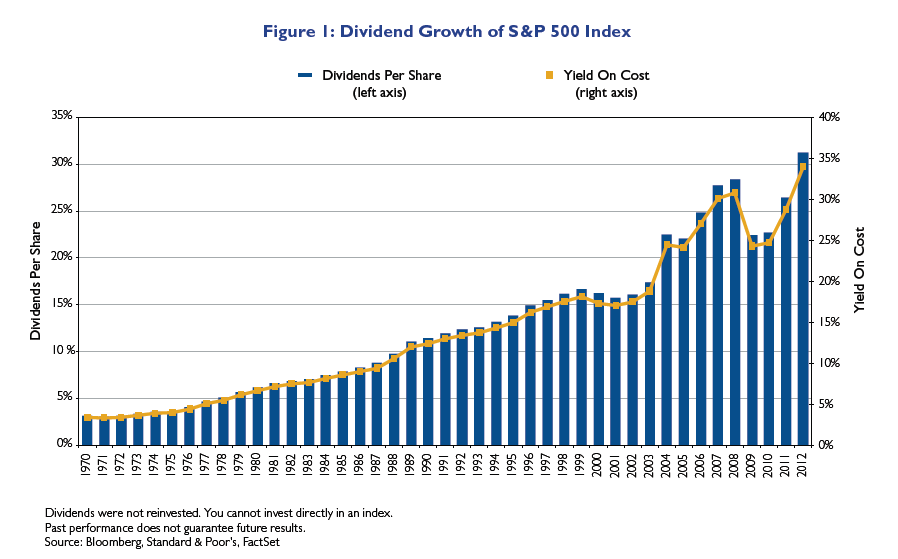# Dividend Growth of S&P 500 From 1972 To 2012

The current yield on the S&P 500 Index is 2.08%. The yield has stayed around the 2% range for many years now. However the dividend amounts paid out components in the index has increased over the years especially in the long run. The following chart shows the dividends paid per share and the yield on cost from 1972 to 2012. The Yield On Cost simply denotes the yield on the cost of investment. So the Yield on Cost on a \$100 investment in a stock paying \$10.0 in year 1 would be 10%. The following year the Yield On Cost would be higher if the dividend paid was lets say \$11.0. If the dividends paid out increases year after year then the Yield On Cost would also increase accordingly.

Click to enlargeSource:The Case for a High and Growing Dividend Stock Strategy in Retirement Portfolios, Thornburg Investments, June 2013

From the Thornburg research study:

Figure 1 assumes if you bought one share of the S&P 500 Index on December 31, 1969, it would have cost you \$92.06. In each subsequent year you chose to spend the dividends rather than reinvest them. In 1970, you would have received dividends totaling \$3.14, for a 3.41 percent yield on cost; by 1980, your annual dividend would have increased to \$6.16,
for a 6.69 percent yield on cost; in 1990 you would have received \$12.09, for a 13.13 percent yield on cost; and in 2012 you would have received \$31.25, for an attractive 33.95 percent yield on cost. In fact, the average annual increase of the dividends over the entire 43-year period was 5.89 percent. (emphasis added)

While the dividend yield is low on the S&P 500, the average annual increase in dividends is decent over the period shown above.

Related ETF:

• SPDR S&P 500 ETF (SPY)

Disclosure: No ETF